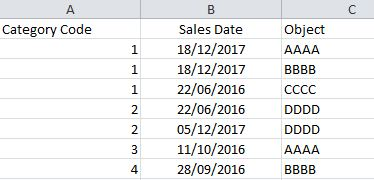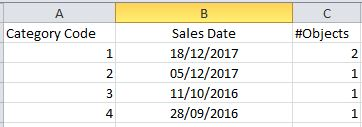# App Development

Announcements
April 22, 2PM EST: Learn about GeoOperations in Qlik Sense SaaS READ MORE
cancel
Showing results for
Did you mean:Partner

## Count Objects for Max Date in Set Analysis

Hello,

I have a table showing Category Code, Sales Date, and Objects:I want to calculate for each Category Code the quantity of distinct Objects that have the max Sales Date for each Category Code:In this example for the Category Code=1, I want that #Objects=2 because there are 2 distinct Objects that have the Sales Date 18/12/2017.

I'm using the Version November 2017 of Qlik Sense.

Any suggestion?

thx a lot,

Arianna

1 Solution

Accepted SolutionsPartner
Author

Hi Hector,

thank u a lot for your reply, but it doesn't function that formula.

After a lot of test I found the solution!Count( Distinct If(Aggr(NODISTINCT Max( TOTAL <[Category Code]> [Sales Date]),[Category Code],[Object]) = [Sales Date], [Object]))

Thanks a lot to everyone,

11 RepliesSpecialist

Count(Aggr(Only(Objects), [Sales Date]))MVP

May be try this

Dimension

Category Code

Measure

Max([Sale Date])

Sum(Aggr(If([Sale Date] = Max(TOTAL <[Category Code]> [Sales Date]), 1, 0), [Category Code], [Sale Date]))Partner

You should try this:

Sales:

"Category Code",

"Sales Date",

Object

FROM [lib://ExcelFilesForTest/Example1.xlsx]

(ooxml, embedded labels, table is Hoja1);

left Join (Sales)

"Category Code",

date(Max("Sales Date")) as MaxDatePerCategory

FROM [lib://ExcelFilesForTest/Example1.xlsx]

(ooxml, embedded labels, table is Hoja1) group by "Category Code";

SalesFinal:

if("Sales Date" = MaxDatePerCategory,1,0) as _flagLastDate

Resident Sales;

drop Table Sales;

Then use this in your Table:

Use Category Code as Dimension, and count({\$<_flagLastDate={1}>} distinct Object)  as mease.

I hope this helps.Partner
Author

Hi Hector,

thank u for your reply, but I can't change the Data Model  (it's more complex than this).

I need a formula that allows the dynamic selection of the Dates or something else that change the quantity of Objects

thank u so much,Partner
Author

Hi Allu,

Thank u for your reply, but unfortunately it doesn't resolve my problem.

I need a formula that calculates for each Category Code how many distinct Objects have the Max Sales Date exists.

thank u so muchPartner
Author

Hi Sunny,

I tested your formula and it works as I expected. In particular that it's ok if I use it in the first Table, because I obtain 1 in the rows where I have the Max Date.

My problem is calculate the count of this 1 in the second Table.

Could you give me another suggestion for this?

thank u a lotMVP

Not entirely sure what you mean? Isn't the 2nd table the expected output of what you are looking to get?Partner
Author

Yes!

I modified your formula and It seems to work. Now I'm doing some test with this:

Sum(TOTAL <[Category Code]> if([Sales Date] = Aggr(max(TOTAL <[Category Code]> [Sales Date]), [Category Code], [Object]), 1, 0))Partner

Hi Arianna,

I know you can not modify your data model, it could be better. This is a modified version of Sunny´s solution and yours:

Try this:

sum(TOTAL <[Category Code]> aggr(if([Sales Date] = max(TOTAL <[Category Code]> [Sales Date]), 1, 0),[Category Code], [Sales Date],[Object]))